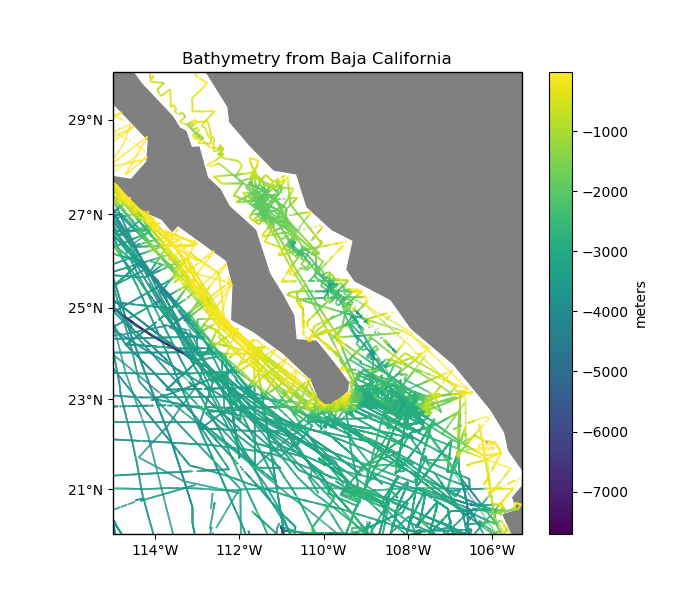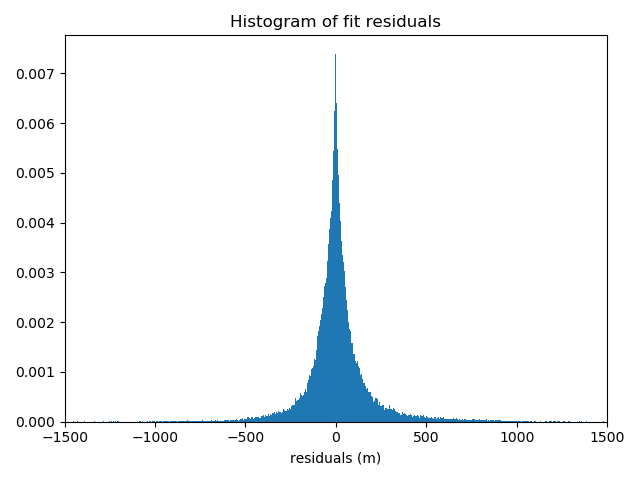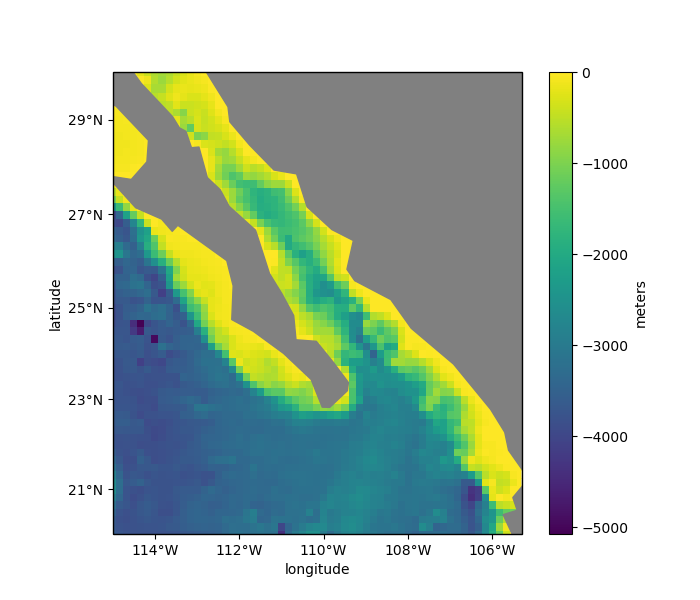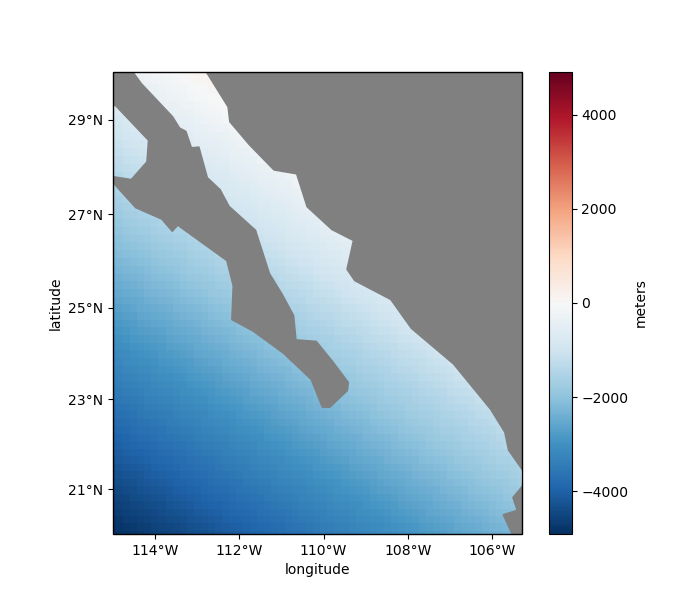# Chaining Operations¶

Often, a data processing pipeline looks like the following:

1. Apply a blocked mean or median to the data

2. Remove a trend from the blocked data

3. Fit a spline to the residual of the trend

4. Grid using the spline and restore the trend

The verde.Chain class allows us to created gridders that perform multiple operations on data. Each step in the chain filters the input and passes the result along to the next step. For gridders and trend estimators, filtering means fitting the model and passing along the residuals (input data minus predicted data). When predicting data, the predictions of each step are added together.

Other operations, like verde.BlockReduce and verde.BlockMean change the input data values and the coordinates but don’t impact the predictions because they don’t implement the predict method.

Note

The Chain class was inspired by the sklearn.pipeline.Pipeline class, which doesn’t serve our purposes because it only affects the feature matrix, not what we would call data (the target vector).

For example, let’s create a pipeline to grid our sample bathymetry data.

import numpy as np
import matplotlib.pyplot as plt
import cartopy.crs as ccrs
import pyproj
import verde as vd

data = vd.datasets.fetch_baja_bathymetry()
region = vd.get_region((data.longitude, data.latitude))
# The desired grid spacing in degrees (converted to meters using 1 degree approx. 111km)
spacing = 10 / 60
# Use Mercator projection because Spline is a Cartesian gridder
projection = pyproj.Proj(proj="merc", lat_ts=data.latitude.mean())
proj_coords = projection(data.longitude.values, data.latitude.values)

plt.figure(figsize=(7, 6))
ax = plt.axes(projection=ccrs.Mercator())
ax.set_title("Bathymetry from Baja California")
plt.scatter(
data.longitude,
data.latitude,
c=data.bathymetry_m,
s=0.1,
transform=ccrs.PlateCarree(),
)
plt.colorbar().set_label("meters")
vd.datasets.setup_baja_bathymetry_map(ax)
plt.tight_layout()
plt.show()Out:

/home/travis/build/fatiando/verde/tutorials/chain.py:57: UserWarning: Tight layout not applied. The left and right margins cannot be made large enough to accommodate all axes decorations.
plt.tight_layout()


We’ll create a chain that applies a blocked median to the data, fits a polynomial trend, and then fits a standard gridder to the trend residuals.

chain = vd.Chain(
[
("reduce", vd.BlockReduce(np.median, spacing * 111e3)),
("trend", vd.Trend(degree=1)),
("spline", vd.Spline()),
]
)
print(chain)


Out:

Chain(steps=[('reduce',
drop_coords=True,
reduction=<function median at 0x7f4998b30620>,
region=None, shape=None, spacing=18500.0)),
('trend', Trend(degree=1)),
('spline',
Spline(damping=None, engine='auto', force_coords=None,
mindist=1e-05))])


Calling verde.Chain.fit will automatically run the data through the chain:

1. Apply the blocked median to the input data

2. Fit a trend to the blocked data and output the residuals

3. Fit the spline to the trend residuals

chain.fit(proj_coords, data.bathymetry_m)


Out:

Chain(steps=[('reduce',
drop_coords=True,
reduction=<function median at 0x7f4998b30620>,
region=None, shape=None, spacing=18500.0)),
('trend', Trend(degree=1)),
('spline',
Spline(damping=None, engine='auto', force_coords=None,
mindist=1e-05))])


Now that the data has been through the chain, calling verde.Chain.predict will sum the results of every step in the chain that has a predict method. In our case, that will be only the Trend and Spline.

We can verify the quality of the fit by inspecting a histogram of the residuals with respect to the original data. Remember that our spline and trend were fit on decimated data, not the original data, so the fit won’t be perfect.

residuals = data.bathymetry_m - chain.predict(proj_coords)

plt.figure()
plt.title("Histogram of fit residuals")
plt.hist(residuals, bins="auto", density=True)
plt.xlabel("residuals (m)")
plt.xlim(-1500, 1500)
plt.tight_layout()
plt.show()Likewise, verde.Chain.grid creates a grid of the combined trend and spline predictions. This is equivalent to a remove-compute-restore procedure that should be familiar to the geodesists among us.

grid = chain.grid(
region=region,
spacing=spacing,
projection=projection,
dims=["latitude", "longitude"],
data_names=["bathymetry"],
)
print(grid)


Out:

<xarray.Dataset>
Dimensions:     (latitude: 61, longitude: 59)
Coordinates:
* longitude   (longitude) float64 245.0 245.2 245.3 ... 254.4 254.5 254.7
* latitude    (latitude) float64 20.0 20.17 20.33 20.5 ... 29.66 29.82 29.99
Data variables:
bathymetry  (latitude, longitude) float64 -3.621e+03 ... 8.798e+03
Attributes:
metadata:  Generated by Chain(steps=[('reduce',\n              BlockReduc...


Finally, we can plot the resulting grid:

plt.figure(figsize=(7, 6))
ax = plt.axes(projection=ccrs.Mercator())
ax.set_title("Gridded result of the chain")
pc = grid.bathymetry.plot.pcolormesh(
ax=ax, transform=ccrs.PlateCarree(), vmax=0, zorder=-1, add_colorbar=False
)
plt.colorbar(pc).set_label("meters")
vd.datasets.setup_baja_bathymetry_map(ax)
plt.tight_layout()
plt.show()Out:

/home/travis/build/fatiando/verde/tutorials/chain.py:126: UserWarning: Tight layout not applied. The left and right margins cannot be made large enough to accommodate all axes decorations.
plt.tight_layout()


Each component of the chain can be accessed separately using the named_steps attribute. It’s a dictionary with keys and values matching the inputs given to the Chain.

print(chain.named_steps["trend"])
print(chain.named_steps["spline"])


Out:

Trend(degree=1)
Spline(damping=None, engine='auto', force_coords=None, mindist=1e-05)


All gridders and estimators in the chain have been fitted and can be used to generate grids and predictions. For example, we can get a grid of the estimated trend:

grid_trend = chain.named_steps["trend"].grid(
region=region,
spacing=spacing,
projection=projection,
dims=["latitude", "longitude"],
data_names=["bathymetry"],
)
print(grid_trend)

plt.figure(figsize=(7, 6))
ax = plt.axes(projection=ccrs.Mercator())
ax.set_title("Gridded trend")
pc = grid_trend.bathymetry.plot.pcolormesh(
ax=ax, transform=ccrs.PlateCarree(), zorder=-1, add_colorbar=False
)
plt.colorbar(pc).set_label("meters")
vd.datasets.setup_baja_bathymetry_map(ax)
plt.tight_layout()
plt.show()Out:

<xarray.Dataset>
Dimensions:     (latitude: 61, longitude: 59)
Coordinates:
* longitude   (longitude) float64 245.0 245.2 245.3 ... 254.4 254.5 254.7
* latitude    (latitude) float64 20.0 20.17 20.33 20.5 ... 29.66 29.82 29.99
Data variables:
bathymetry  (latitude, longitude) float64 -4.911e+03 ... 2.264e+03
Attributes:
metadata:  Generated by Trend(degree=1)
/home/travis/build/fatiando/verde/tutorials/chain.py:158: UserWarning: Tight layout not applied. The left and right margins cannot be made large enough to accommodate all axes decorations.
plt.tight_layout()


Total running time of the script: ( 0 minutes 9.046 seconds)

Gallery generated by Sphinx-Gallery